Power Torque continues to be a leading integrator in the motion control and electrical / mechanical power transmission fields. Let our combined experience of over 400 years design a solution for you.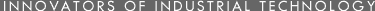• •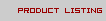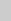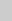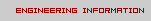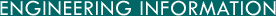N O P Q R

N

• Nail (Br) = 2.25 inches, 5.715 centimeters.

• Newton (force)
= 1x10^5 Dyne, 0.22481 pounds weight. (Assumes G=980.665 cm / second squared.)

• Noggin (Br)
= 1/32 liquid gallon, 142.06 cubic centimeters.

O

• Ounce (fluid US)
= 1/128 gallon, 0.3125 liquid quart, 1/16 liquid pint, ¬ gill, 1.80469 cubic inches, 8 fluid drams, 480 minims, 0.0295729 liter, 0.295729 decaliter, 29.5729 milliliters, 29.5737 cubic centimeters.

• Ounce (fluid Br)
= 0.006250 Br gallon, 8 fluid Br drachms, 480 minims, 28.4130 cubic centimeters.

• Ounce (avoirdupois)
= 2.790179x10^-5 long ton, 3.125x10^-5 short ton, 6.25x10^-4 short hundredweight, 0.075954861 apothecary or troy pound, 1/16 avoirdupois pound, 0.9114583 apothecary or troy ounce, 7.29166 apothecary or troy drams, 16 avoirdupois drams, 18.22917 pennyweights, 21.875 apothecary scruples, 437.5 grains, 2.83495x10^-5 metric ton, 28.349527 grams.

• Ounce (apothecary or troy)
= 3.4285x10^-5 short ton, 0.06857143 avoirdupois pound, 0.08333 apothecary or troy pound, 1.09714 avoirdupois ounces, 17.55428 avoirdupois drams, 8 apothecary or troy drams, 20 troy pennyweights, 24 scruples, 480 grains, 31.103481 grams, 3.1103481x10^4 milligrams.

P

• Pace
= 2.5 feet, 30 Br inches, 76.2 centimeters.

• Palm (Br)
= 3 inches, 7.62 centimeters.

• Parsec
= 1.92x10^13 miles, 3.26 light years, 3.084x10^13 kilometers.

• Parts Per Million (PPM)
= 0.0584 grains per gallon, 0.07016 grains per Br gallon, 8.345 pounds per million gallons.

• Pascal
= 9.86923x10^-6 Standard Atmospheres, 1.4515x10^-4 psi, 0.0102 cm water.

• Peck
= 0.25 bushel, 8 quarts, 16 pints, 537.605 cubic inches, 0.880958 dekaliter, 8.80958 liters.

• Peck (Br)
= 2 Br gallons, 554.6 cubic inches, 9.90919 liters.

• Pennyweight (troy)
= 0.003428571 avoirdupois pounds, 0.0041667 apothecary or troy pound, 1/20 apothecary or troy ounce, 0.0548571 avoirdupois ounce, 0.8777143 avoirdupois dram, 24 grains, 1.55517 grams, 1555.17 milligrams.

• Perch (US & Br)
= 1 rod, 16.5 feet, 5.0292 meters.

• Perch (masonry)
= 24.75 cubic feet.

• Pica
= 1 Em, 1/6 inch, 0.42333 centimeter.

• Pied (Fr)
= 1/6 Fr toise, 12 Paris inches, 0.3248 meter.

• Pint (dry)
= 1/64 bushel, 0.0625 peck, 0.5 quart, 33.600 cubic inches, 0.550599 liter, 550.61 cubic centimeters.

• Pint (liquid)
= 6.1881x10^-4 cubic yard, 0.016711 cubic foot, 0.125 gallon, 0.5 quart, 0.83268 Br pint, 4 gills, 16 fluid ounces, 28.875 cubic inches, 128 fluid drams, 7680 minims, 0.473167 liter, 473.167 milliliters, 473.179 cubic centimeters.

• Pint (Br liquid)
= 0.125 Br gallon, 0.5 Br quart, 1.20094 US pint, 4 Br gills, 20 Br fluid ounces, 0.56825 liter, 568.25 milliliters, 568.26 cubic centimeters.

• Point (printer's type)
= 1/72 inch, 0.35278 centimeters.

• Poise (viscosity)
= 1 gram per centimeter per second, .0010197 gram weight second per square centimeter, .002092 pound weight second per square foot, 1.45032x10^-5 reyn, 100 centipoises.

• Pole (Br)
= 1 rod, 5.5 yards, 16.5 feet, 5.0292 meters.

• Pottle (Br)
= 0.5 liquid gallon, 2.273 cubic decimeters.

• Pouce (Paris inch)
= 1/12 pied, 12 lignes (Paris lines), 2.70700 centimeters.

• Pound (avoirdupois)
= The mass of 27.692 cubic inches of water weighed in air at 4 degrees C and 760 mm pressure, 4.464286x10^-4 long ton, 5.0x10^-4 short ton, 0.0089286 long hundredweight, 0.01 short hundredweight, 1.2152778 apothecary or troy pounds, 16 avoirdupois ounces, 14.5833 apothecary or troy ounces, 256 avoirdupois drams, 116.6667 apothecary or troy drams, 291 6667 pennyweights, 350.01 scruples, 7000 grains, 4.5359243x10^-4 metric ton, 0.4535924 kilogram, 453.5924 grams.

• Pound (apothecary or troy)
= 3.6735x10^-4 long ton, 4.1143z10^-4 short ton, 0.822857 avoirdupois pound, 12 apothecary or troy ounces, 13.165714 avoirdupois ounces, 96 apothecary or troy drams, 210.6514 avoirdupois drams, 240 pennyweights, 288 scruples, 5760 grains, 3.7324x10^-4 metric ton, 0.3732418 kilogram, 373.2418 grams.

• Poundal (force)
= 0.031081 pound weight, 14.098 grams weight, 1.3825x10^4 dynes. (Assumes G=980.665 cm / second squared).

• Puncheon (Br)
= 70 Br gallons, 84 wine gallons, 0.31823 cubic meter.

Q

= 1.57080 radians, 90 degrees, 5400 minutes.

• Quart (dry)
= 1/32 bushel, 0.038889 cubic foot, 0.125 peck, 2 dry pints, 67.2006 cubic inches, 1.10120 liters, 1101.23 cubic centimeters.

• Quart (liquid)
= 0.033421 cubic foot, 0.25 gallon, 2 liquid pints, 8 gills, 32 fluid ounces, 57.749 cubic inches, 256 fluid drams, 0.946333 liters, 946.358 cubic centimeters.

• Quart (Br liquid)
= 0.25 Br gallon, 2 Br liquid pints, 1.13650 liters, 1136.52 cubic centimeters.

• Quarter (US mass)
= 0.25 short ton, 500 pounds, 226.795 kilograms.

• Quarter (US mass)
= 0.25 long ton, 560 pounds, 254.01 kilograms.

• Quarter (Br capacity)
= 8 bushels, 2.909 hectoliters.

• Quarter (Br linear)
= 1 span, 0.25 yard, 9 inches, 22.86 centimeters.

• Quarter (Br mass)
= 0.25 short hundredweight, 25 pounds, 11.340 kilograms.

• Quarter (Br mass)
= 0.25 long hundredweight, 28 pounds, 12.70 kilograms.

• Quartern (Br dry)
= 0.5 gallon, 2273.1 cubic centimeters.

• Quartern (Br liquid)
= 1/32 gallon, 142.07 cubic centimeters.

• Quintal (metric)
= 1.96841 long hundredweights, 220.46 pounds, 100 kilograms, 1x10^5 grams.

• Quintal (US or Br)
= 100 or 112 pounds (variable).

• Quintal (Argentine)
= 101.28 pounds.

• Quintal (Brazil)
= 129.54 pounds.

• Quintal (Chile)
= 101.41 pounds.

• Quintal (Mexico)
= 101.47 pounds.

• Quint. (Castile, Peru)
= 101.43 pounds.

• Quire
= 25 sheets.

R

= 1/(2Pi), 0.159155 circumference or revolution, 0.637 quadrant, 57.29578 degrees, 57 degrees - 17 minutes - 44.8 seconds, 3437.75 minutes, 2.06265x10^5 seconds, 1x10^3 angular mils.

• Ream
= 500 sheets.

• Register Ton (Br)
= 100 cubic feet, 2.8317 cubic meters.

• Revolution

• Reyn (viscosity)
= Pounds force seconds per square inch, 6.895x10^4 poise.

• Rod (Surveyors measure)
= 0.003125 mile, 0.025 furlong, 0.25 Gunter's chain, 1 perch, 5.5 yards, 16.5 feet, 25 links, 198 inches, 5.029210 meters.

• Rod (Br volume)
= 1000 cubic feet, 28.317 cubic meters.

• Rood (Br)
= 0.25 acre, 40 square perches, 1210 square yards, 10.117 ares or square dekameters.

• Rope (Br)
= 20 feet, 6.0960 meters.